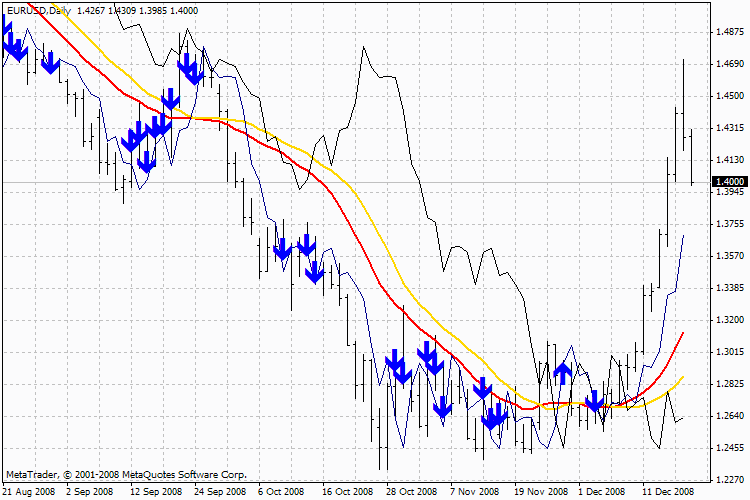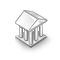それではリンクにそれを投稿してください。-# Ryan_Jones_SM - MetaTrader 4のためのインディケーター

ビュー:
13104
レーティング:

パブリッシュされた:
2008.12.25 07:12
アップデートされた:
2016.11.22 07:32

It shows where to buy and where to sell via the arrows. If a trend occurs then it will bring profit, if there is no trend, there will be no profit.

Input Parameters:

```extern int       ParY=3;  //"Y" parameter
extern int       ParX=20; //"X" parameter```

### Rules:

The method that gives those numbers is miraculously simple. The rules are following:

Buy:
1. The average close value calculated for the "X"-days period should be greater than the analogous average value calculated for past "Y" days.
2. The close price should be less than the analogous price "Y" days ago.
3. The close price should be greater than the close price "Y+X" days ago.

If all three conditions are fulfilled then you should by at the opening of the next day

Sell:
1. The average value calculated by closing for "X"-days period should be less than the analogous average value calculated for past "Y" days.
2. The close price should be greater than the analogous price "Y" days ago.
3. The close price should be less than the close price "Y+X" days ago.

For example, if "Х"= 20 and "Y"=3Ryan_Jones_SMMultiNeyro

The algorithm of tuning (optimization) by two ranges with the two-level three-stage optimization is used in the EA.MiniVirtualAccount

Running an EA in a virtual accountBurg Extrapolator

The EA uses the method of Burg's linear prediction that was taken from my indicator Extrapolator.mq4.Extrapolator

Extrapolator is a result of my long-term research in the area of the Timeseries Forecasting.